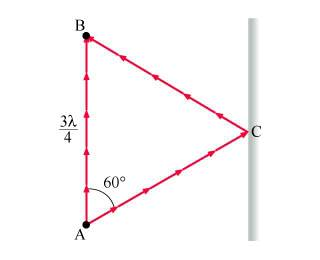## Homework Statement

Consider two light rays propagating from point A to point B in the figure, which are 3(lambda)/4 apart. One ray follows a straight path, and the other travels at a 60 degree angle to that path and then reflects off a plane surface to point B. Both rays have wavelength lambda. View FigureFind the phase difference between these two rays at point B. Answer in terms of pi.

## Homework Equations

No equations were provided. But according to my handy textbook:

Phase Difference = 2(pi)(change in x)/(lambda) + change in initial phase

## The Attempt at a Solution

(Phase Difference) = 2(pi)[3lambda/4lambda] + (60 degrees * 2pi/360 degrees)
= 1.83pi

any ideas?

first of all u havent understood really what phase difference is....
it has nothing to od with angle between the rays...
study some more about phase difference articls on net or yourhandy textbook
so 60 is not initial phase diff
as initial is not given we can take it as zero is uppose
next
change in x is difference in path travelled by the two rays....
here 1 ray travels 3lambda /4
now find the path travelled by the other ray by considering the triangle and laws of reflection
i =r

you will find the triangle to be equilateral
so change in x = 2X 3lambda/4 - 3lambda/4=3lambda/4
i hope no problems...but you got some conceptual probs go figure them out